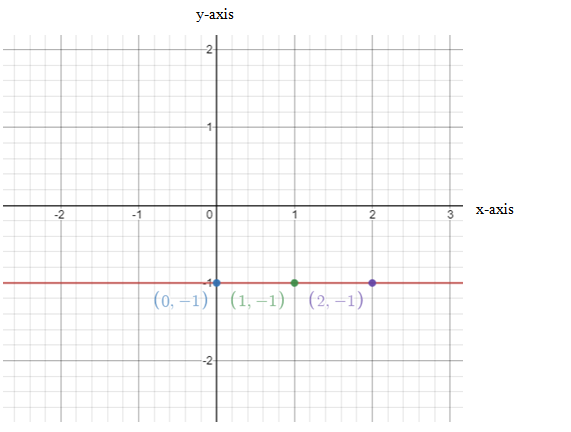# To sketch the graph of the equation### Single Variable Calculus: Concepts...

4th Edition
James Stewart
Publisher: Cengage Learning
ISBN: 9781337687805### Single Variable Calculus: Concepts...

4th Edition
James Stewart
Publisher: Cengage Learning
ISBN: 9781337687805

#### Solutions

Chapter B, Problem 8E
To determine

## To calculate: To sketch the graph of the equation

Expert Solution

The graph of equation is sketched

### Explanation of Solution

Given information: Equation is y=2

Calculation:

Equation is given as follows:

y=2

Finding the points that lie on equation:

When x=0

y=2

When x=1

y=2

When x=2

y=2

Thus, (0,1),(1,1),(2,1) are the solution of equation and must lie on the equation.

Plotting the points on graph,Conclusion:

Hence, graph of equation is sketched

### Have a homework question?

Subscribe to bartleby learn! Ask subject matter experts 30 homework questions each month. Plus, you’ll have access to millions of step-by-step textbook answers!